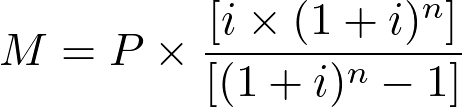# mortgager - R PackageMortgage Calculator Formula

# {mortgager}

This package was developed to calculate homeownership costs for MassHousing’s Commonwealth Builder Program. This package was used to analyse a proprietary dataset of home sale records from 2010 to 2020 in Massachusetts to determine the affordability of existing and historic sales.

## Formula

The formula used for calculating monthly home-ownership payment amounts is based on a widely accepted monthly mortgage formula, which here is adapted from that seen on the Nerdwallet website.Where:

• M = Monthly mortgage amount
• P = Principal amount, ie total loan amount
• i = Monthly mortgage interest rate
• n = Number of payments across loan duration. (This is generally assumed to be number of months. For example, a loan with a payback period of 30 years, will have 360 payments in total)

In addition to the monthly mortgage payment, there are other costs associated with home-ownership which are then added to calculate total home-ownership costs, namely:

• PMI factor – only for loans with a downpayment less than 20%, assumed at 7.5% annually on the mortgage amount
• Home-owners insurance – assumed at \$1000 annually
• Condo fees – applicable only to condominium sales, assumed at \$1000 annually
• Property Tax - an annual percentage amount taxed on assessed property value dependent on the jurisdiction the unit is located in.

## Installation

You can install the released version of mortgager with:

``devtools::install_github("aseemdeodhar/mortgager")``

## {mortgager}

Load the package with this line:

``library(mortgager)``

## Examples for each function:

### mortgager::monthlypayment()

calculates monthly home-ownership costs based on tax rates, interest rates, downpayment rate, and home cost price.

``````monthlypayment(mortgage_rate = 3,
property_tax = 12,
downpayment_rate = .2,
home_price = 450000)``````
``##  2051.108``

### mortgager::max_homeprice()

calculates maximum affordable home price based on annual income with a set monthly housing cost percentage (default is 30%).

``````max_homeprice(annualinc = c(61000, 75000, 22330, 120000),
mortgage_rate = 2.7,
property_tax = 6)``````
``````## # A tibble: 4 x 4
##   income_level atincome  at80pc at120pc
##          <dbl>    <dbl>   <dbl>   <dbl>
## 1        61000  224144. 179316. 268973.
## 2        75000  278561. 222849. 334273.
## 3        22330   73838.  59070.  88606.
## 4       120000  453471. 362777. 544166.``````

### mortgager::downpayment_amt()

calculates the total downpayment amount based on sale price and downpayment rate

``````downpayment_amt(home_price = 250000,
downpayment_rate = 0.10)``````
``##  25000``

### mortgager::reqd_income()

calculates the minimum annual income required for total housing costs to remain at or below a set proportion of household income.

``````reqd_income(monthlypayment = 2000,
housing_cost_rate = 0.30)``````
``##  80000``##### Aseem Deodhar
###### Research Analyst I

My research interests include transit user behaviour, spatial movement pattern analysis, and transportation infrastructure.